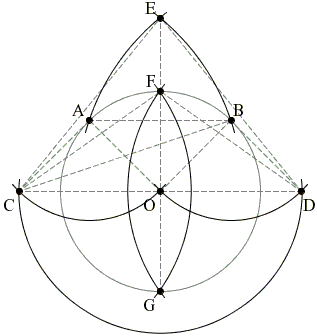# Bisect an Arc

### Geometric Construction with the Compass Alone

Let two points A and B belong to a circle with center O. Bisect the two arcs of the circle defined by the points A and B.

## SolutionStart with completing two parallelograms (Problem #6): ABOC (C is opposite to B) and OABD (D is opposite to A). Draw two circles with radius r = AD = BC: one centered at C, another at D. Let E be one of the intersection points. Finally, circles with the radius OE and centers at C and D intersect at the midpoints F and G of the two arcs AB.

Note that the arcs AB do not have to be actually drawn.

## Proof

Let R be the radius of the original circle. By construction, the points C, D and O are collinear (lie on the same straight line.) The same is true for the points F, G and O. Furthermore, OF is perpendicular to CD. All we have to show is that OF = R.

For a parallelogram XYZW we have the following identity

 XZ² + YW² = 2(XY² + YZ²)

which I'll apply to the parallelogram ABOC to get r² = 2AB² + R². Since the triangle COE is right-angled, r² = CE² = EO² + CO², by the Pythagorean Theorem. Therefore, OE² = AB² + R². However, the triangle COF is also right-angled wherefrom OC² + OF² = CF² = OE². Combining the last two identities we get OF = R. Q.E.D.

A rather specialized problem [Trigg, #248] asks to divide a circle with a known radius into four equal parts. The problem is easily solved by first finding two diametrically opposite points and then applying the above construction to the semicircles so defined. Starting with an arbitrary point A, mark on the circle points C, D, B such that the distances AC, CD, DB all equal the radius of the circle. Point B is opposite A on the circle, so that AB is a diameter.

### Reference

1. C. W. Trigg, Mathematical Quickies, Dover, 1985### Problems (Use a compass only)